# Get the Current Workbook Name

This post explains how to get the current workbook name in excel.

## Get the Current Workbook Name

In excel, the CELL function can get the information of the current worksheet, it will return the full file name and its path. If you want to get the name of the current workbook only, you can use a combination of the MID function, the CELL function and the FIND Function. Just refer to the below generic formula:

`=MID(CELL("filename",B1),FIND("[",CELL("filename",B1))+1,FIND("]",CELL("filename",B1))- FIND("[",CELL("filename",B1))-1)`

Let’s see how the above formula works:

The MID function will extract a sub-string from a text string at the position that you specify. It contains three arguments in MID function.

The first argument is the returned results of the first CELL function. The full workbook name and its path will be returned from the below CELL function.

`=CELL("filename",B1)`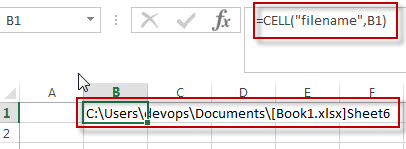The first FIND function will locate the position of the first bracket along with the workbook name. It is the second arguments as the MID function.

`=FIND("[",CELL("filename",B1))`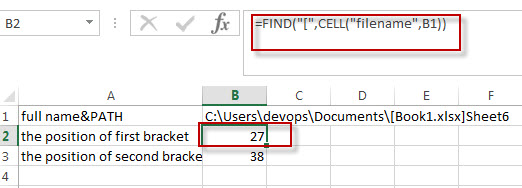The second FIND function will return the position of the second bracket right of the workbook name.

`=FIND("]",CELL("filename",B1))`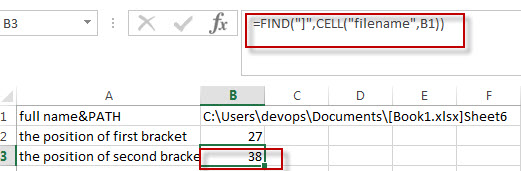The third argument of the MID function is the length of the substring that you want to extract. In other words, you need to get the length of the workbook name. Using the below formula:

`=FIND("]",CELL("filename",B1))- FIND("[",CELL("filename",B1))-1`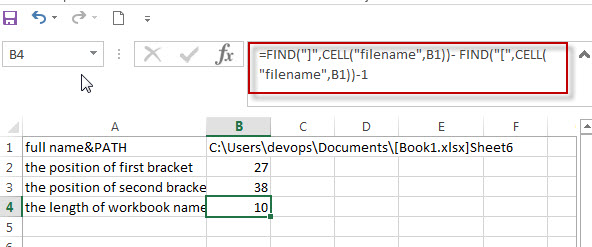Last, we can run the following excel formula to get the current workbook name:

`=MID(CELL("filename",B1),FIND("[",CELL("filename",B1))+1,FIND("]",CELL("filename",B1))- FIND("[",CELL("filename",B1))-1`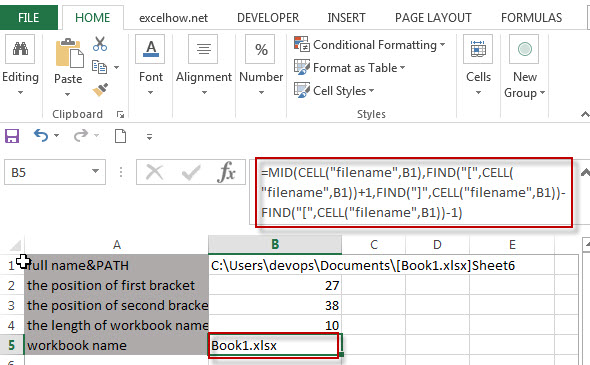### Related Functions

• Excel Find function
The Excel FIND function returns the position of the first text string (substring) from the first character of the second text string.The FIND function is a build-in function in Microsoft Excel and it is categorized as a Text Function.The syntax of the FIND function is as below:= FIND  (find_text, within_text,[start_num])…
• Excel MID function
The Excel MID function returns a substring from a text string at the position that you specify.The syntax of the MID function is as below:= MID (text, start_num, num_chars)…
• Excel CELL function
The Excel CELL function returns information about the formatting, location, size, or contents of a cell.The syntax of the CELL function is as below:= CELL (info_type,[reference])…